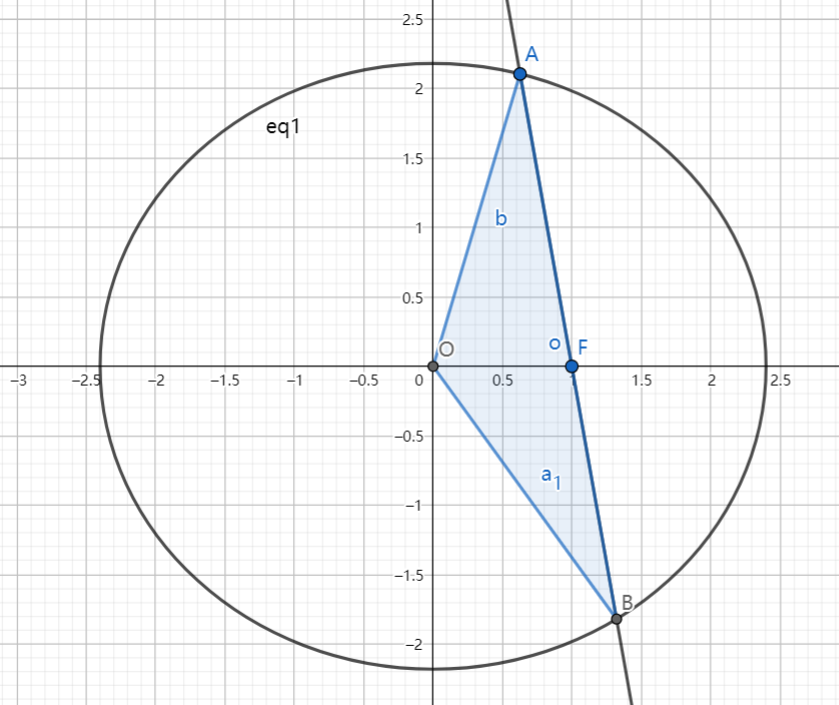# SAT1000 - P819

Geometry Level pendingAs shown above, given that $F(1,0)$ is the right focus of the ellipse: $\dfrac{x^2}{a^2}+\dfrac{y^2}{b^2}=1\ (a>b>0)$, $O$ is the origin.

If for all lines passing through $F$ which intersect with the ellipse at point $A,B$, the following inequality always holds:

$|OA|^2+|OB|^2<|AB|^2$

Then find the range of $a$.

If the range can be expressed as: $(l,+\infty)$, submit $\lfloor 1000l \rfloor$.

Have a look at my problem set: SAT 1000 problems

×